1631607049

# The Breadth First Search (BFS) Algorithm | Visualized and Explained

In this video we break down the BFS algorithm in a visual manner with examples and key intuition. We then show the implementation of the algorithm with code and then finish off the video by demonstrating how you can use the BFS algorithm to solve the Flood Fill problem.

0:00 Introduction
0:45 BFS Intuition/Examples
2:39 BFS Implementation
5:19 Flood Fill Problem

This video wouldn't be possible without the open source manim library created by 3blue1brown: https://github.com/3b1b/manim

Here is link to the repository that contains the code used to generate the animations in this video: https://github.com/nipunramk/Reducible

## Buddha Community1596737580

## Graphs Data Structure: Breadth First Search

A week ago we learned about graph data structure. Today we will talk about how we can work with graphs. We will try to find distances between two nodes in a graph. This is one of the main uses of graphs and it’s called graph traversal. There are two main graph algorithms Breadth First Search (BFS) and Depth First Search (DFS) and today we will talk about BFS.

This is how our graph looks like: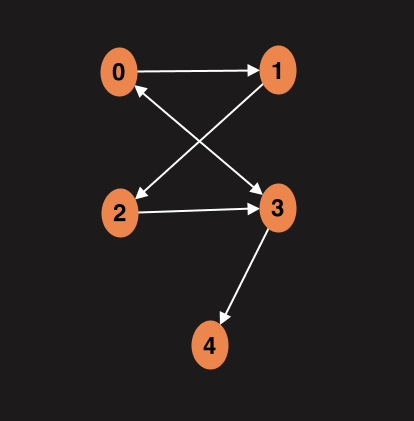In our example we will work with an adjacency matrix. This is how matrix represents graph above: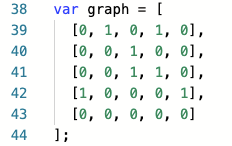We will start with an input node, then visit all its neighbors which is one edge away. And then visit all their neighbors. Point is to determine how close the node is to the root node.

Function which we will write in a moment will return an object with key value pairs where key will represent node and value how far this node is from the root.

First we will loop over the adjacency matrix (2D array), create as many key value pairs as many nodes we have on the graph. Initially we will assign distance to the infinity which represents lack of connection between the nodes.

#graph #data-structures #breadth-first-search #javascript #algorithms #algorithms1603767600

## Search Algorithms

Today, let us touch base on some fundamental concepts like search algorithms.

In simple terms, **searching **is a process of looking up a particular data record in the database or in the collection of items. A search typically answers as true or false whether the particular data in which we are referring is found or not and perform the next action accordingly.

Commonly used algorithms for search are:

• Linear search
• Binary search
• Interpolation search

Let us understand them in detail with some example

## Linear Search Algorithm

Linear Search Algorithm is the simplest and easiest form of the search algorithm. In this algorithm, a sequential search is made over all the items one by one to search for the targeted item. Each item is checked in sequence until the match is found. If the match is found, the searched item is returned otherwise the search continues till the end.

To make it easy to understand, let us see understand linear search using a flow diagram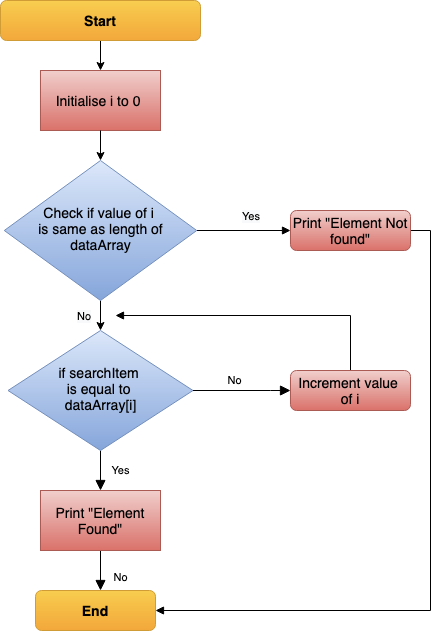Linear Search — Data Flow

### Points to note:

• Does not need sorted array list
• Performs equality comparisons
• The time complexity is O(n)
• Time taken to search elements keeps increasing as the number of elements is increased.

## Binary Search Algorithm

In _Binary search algorithm, _begins with an interval covering the whole array and diving it in half. If the value of the search key is less than the item in the middle of the interval, narrow the interval to the lower half. Otherwise narrow it to the upper half. Repeatedly check until the value is found or the interval is empty.

To make it easy to understand, let us see understand binary search using flow diagram and example as below.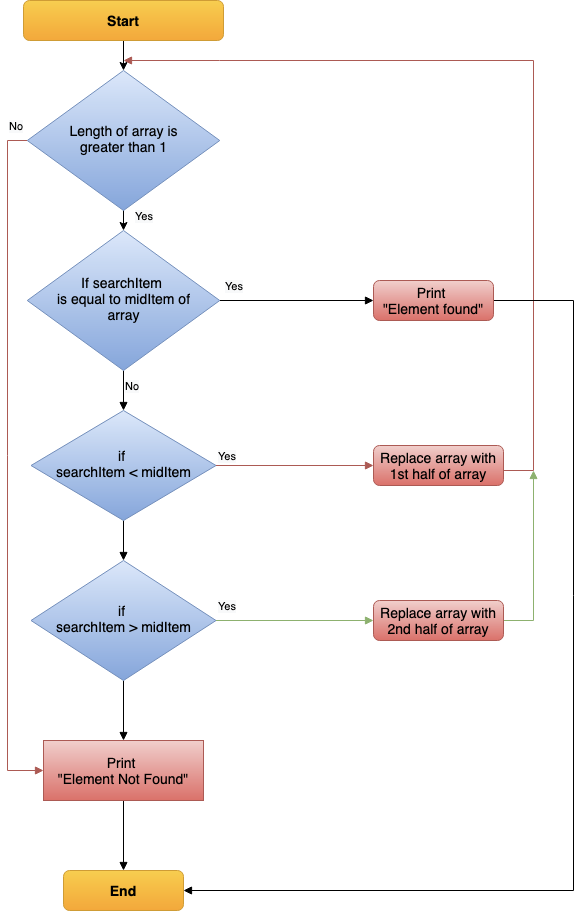Binary Search — Data Flow

### Points to note:

• The array needs to be sorted
• Performs ordering comparisons
• Time complexity to O(log n).
• Search is done to either half of the given list, thus cut down your search to half time

#sorting-algorithms #algorithms #data-structures #search-and-sort #searching-algorithm1592844780

## Depth First Search (DFS) Algorithm Visually Explained

The Depth First Search algorithm traverses the graph and explores each adjacent node before backtracking and moving to the next node. It utilizes the stack data structure. Remember, the stack uses the last-in-first-out (LIFO) approach. We push items onto the stack and pop items from the top of the stack. We’ll keep track of the stack and we’ll show the vertices in the order of which they were discovered.
We’ll begin our traversal at vertex A. Vertex A has been discovered, so it’s added to the discovery list. Vertex A is also pushed onto the stack and is marked as visited.

#dfs #algorithms #programming #depth-first-search #computer-science1596737760

# Statement

We have to search for a value **x **in a sorted matrix M. If **exists, then return its coordinates **(i, j), else return (-1, -1).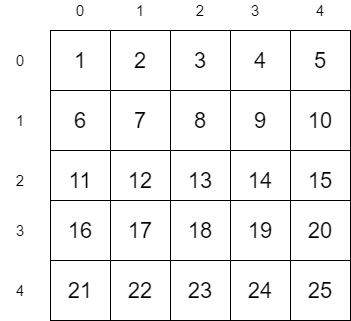Let us consider the above matrix as an example. In this example, we are going to search for the value 12. Since 12 is present in the matrix, the algorithm should return its coordinates (2, 1)

# Simple Approach

A simple approach is to traverse all the values in the matrix and check if it is equal to 12.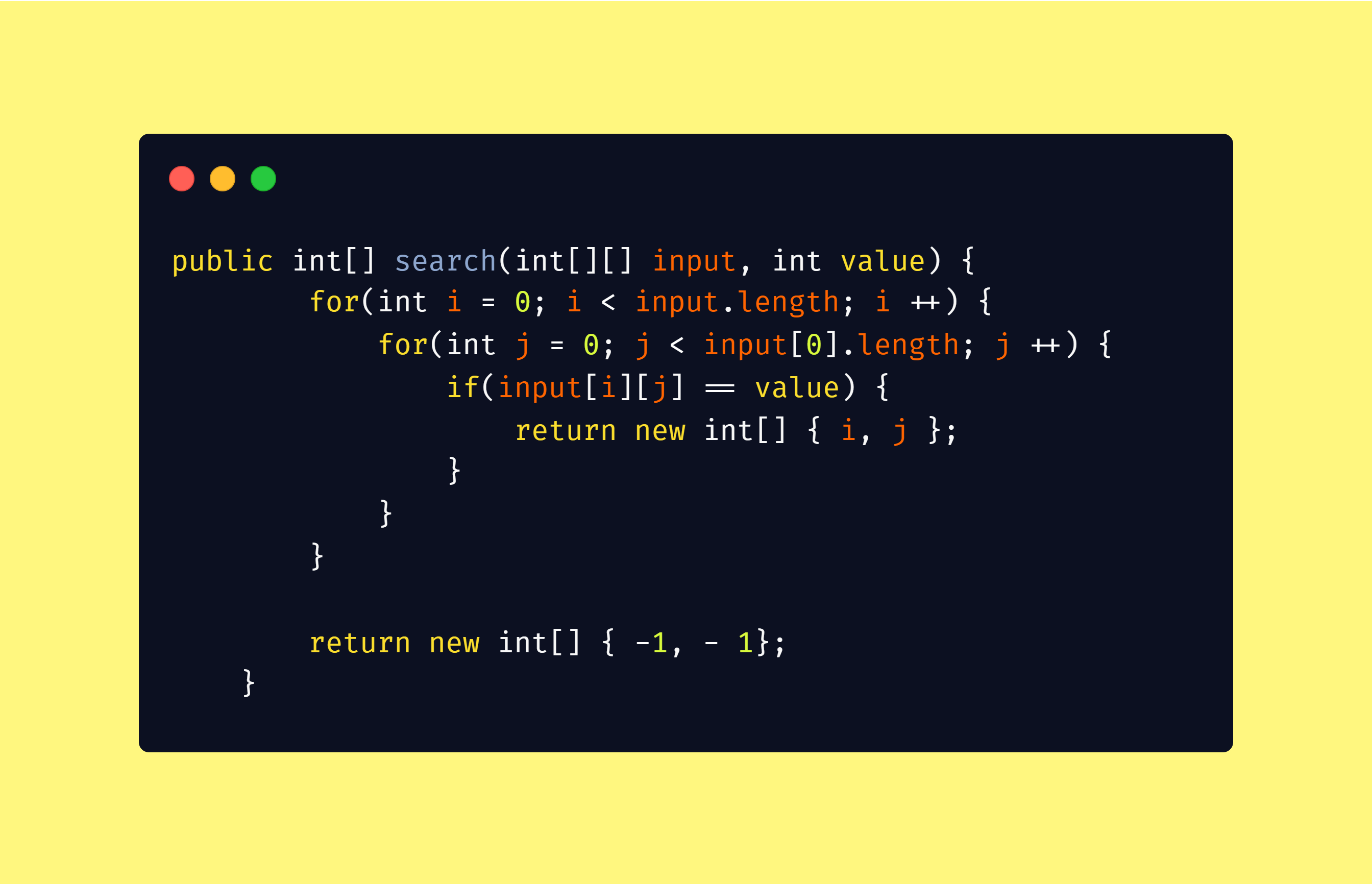The worst case time complexity of the above algorithm will be

_O(n x m) = O(n²) __when _n = m

# Efficient Approach

The above algorithm behaves worse for large values of n and m. Let us look into the efficient algorithm now.

## Algorithm

``````1\. Start from Top Right position (0, m - 1) in the matrix M
2\. If the value is equal to x return (0, m - 1)
3\. Move one row down if the current value is less than x
4\. Move one column left if the current value is greater than x
``````

Let us apply this algorithm into our matrix M. We are going to search for the value 12 in M

#data-structures #search-sorted-matrix #2d-binary-search #algorithms #matrix-search #algorithms1597045745

## Searching Algorithms in Java

Searching Algorithms are designed to check for an element or retrieve an element from any data structure where it is stored. Based on the type of search operation, these algorithms are generally classified into two categories:

1. Sequential Search: In this, the list or array is traversed sequentially and every element is checked. For Example: Linear Search.
2. Interval Search: These algorithms are specifically designed for searching in sorted data-structures. These type of searching algorithms are much more efficient than Linear Search as they repeatedly target the center of the search structure and divide the search space in half. For Example: Binary Search.

Linear Search: The idea is to traverse the given array arr[] and find the index at which the element is present. Below are the steps:

• Let the element to be search be x.
• Start from the leftmost element of arr[] and one by one compare x with each element of arr[].
• If x matches with an element then return that index.
• If x doesn’t match with any of elements then return -1.

#java #searching #algorithms-searching #binary search